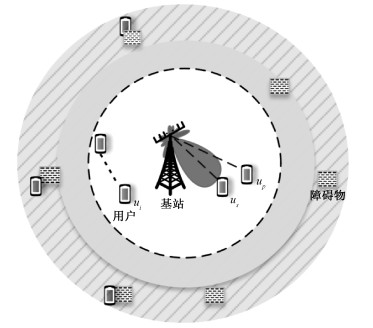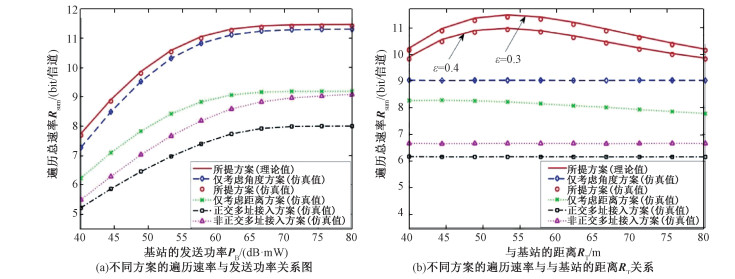﻿ 毫米波网络非正交多址接入的新型用户配对方案
 中国科学院大学学报2022, Vol. 39Issue (2): 252-259PDF

1. 上海科技大学信息科学与技术学院, 上海 201210;
2. 中国科学院上海微系统与信息技术研究所, 上海 200050;
3. 中国科学院大学, 北京 100049

A novel user pairing scheme for millimeter wave non-orthogonal multiple-access networks
LU Xiaolin1,2,3, ZHOU Yong1
1. School of Information Science and Technology, ShanghaiTech University, Shanghai 201210, China;
2. Shanghai Institute of Microsystem and Information Technology, Chinese Academy of Sciences, Shanghai 200050, China;
3. University of Chinese Academy of Sciences, Beijing 100049, China
Abstract: For the features of high-speed and multiple-requirements of multiple users in 5G millimeter wave networks, in order to further enhance the downlink non-orthogonal multiple-access transmission in millimeter wave networks, a novel user pairing scheme based on angle and distance is proposed in this paper. This scheme fully considers the high directional beam-forming of the antenna array and the randomness of the user. The base station will choose to enable the nonorthogonal multiple access scheme based on the instantaneous spatial angle difference and the distance information between the paired users. Using methods from stochastic geometry, we derive the ergodic sum rate of the proposed scheme. Simulation results show that this scheme outperforms four other common user pairing schemes, and there is an optimal value of the distance threshold that maximizes the ergodic sum rate.
Keywords: millimeter wave    non-orthogonal multiple-access(NOMA)    user pairing    stochastic geometry

1 系统模型Download: JPG larger image 图 1 系统拓扑模型图 Fig. 1 Topology of the system model

 $\boldsymbol{a}\left(\vartheta_{i}\right) = \frac{1}{\sqrt{N}}\left(1, \cdots, \mathrm{e}^{\mathrm{j} 2 {\rm{ \mathsf{ π} }} k \rho \vartheta_{i}}, \cdots, \mathrm{e}^{\mathrm{j} 2 {\rm{ \mathsf{ π} }}(N-1) \rho \vartheta_{i}}\right)^{\mathrm{T}}.$ (1)

 $G\left(\vartheta_{i}-\theta\right) = \frac{\sin ^{2}\left({\rm{ \mathsf{ π} }} \rho N\left(\vartheta_{i}-\theta\right)\right)}{N^{2} \sin ^{2}\left({\rm{ \mathsf{ π} }} \rho\left(\vartheta_{i}-\theta\right)\right)} = \frac{\sin ^{2}\left({\rm{ \mathsf{ π} }} \rho N \varphi_{i}\right)}{N^{2} \sin ^{2}\left({\rm{ \mathsf{ π} }} \rho \varphi_{i}\right)}.$ (2)

2 新型的非正交多址接入传输策略 2.1 匹配用户的选择准则

 $x_{p} = \arg \min \limits_{x_{i} \in \Phi\left(R_{T}\right) \backslash\left\{x_{s}\right\}} \varphi_{i s}.$ (3)

2.2 新型传输策略的分析

NOMA传输    在情况1中，首先解码用户up的信号。基站调整其朝向用户us的波束方向。因此，基站和用户us之间的阵列增益为NG(0)，由于G(0)=1，因此等于N。传输给用户up的信号并由用户us解码的信号与干扰加噪声比(SINR)为

 $\varGamma_{p \rightarrow s} = \frac{\alpha_{p} N P_{B}\left|h_{s}\right|{ }^{2} l\left(r_{s}\right)}{\alpha_{s} N P_{B}\left|h_{s}\right|^{2} l\left(r_{s}\right)+\sigma^{2}},$ (4)

 $\varGamma_{s} = \alpha_{s} N P_{B}\left|h_{s}\right|^{2} l\left(r_{s}\right) / \sigma^{2},$ (5)

 $\varGamma_{p | s} = \frac{\alpha_{p} N P_{B}\left|h_{p}\right|^{2} l\left(r_{p}\right) G\left(\varphi_{p s}\right)}{\alpha_{s} N P_{B}\left|h_{p}\right|^{2} l\left(r_{p}\right) G\left(\varphi_{p s}\right)+\sigma^{2}},$ (6)

OMA传输    发生情况2或情况3时，将启用OMA传输方案。基站调整其波束方向以使其自身与用户us之间的信道矢量对准。因此，在用户ui，其中i∈{s, p}处观察到的SNR为

 $\varGamma_{i}^{\mathrm{OMA}} = N P_{B}\left|h_{i}\right|^{2} l\left(r_{i}\right) / \sigma^{2}.$ (7)

3 用户遍历总速率分析

 $f_{r_{s}}(r) = 2 K r R_{T}^{-2}\left(1-r^{2} / R_{T}^{2}\right)^{K-1}, 0 基于提出的用户配对策略，用户up必须与用户us不是同一个，并且其到基站的距离应大于其到用户us的距离，即rp>rs。如果存在配对的用户，则基站与用户之间距离rp的条件概率密度函数为 $ f_{r_{p} \mid r_{s}}(r) = \left\{\begin{array}{cc} 2 r\left(R_{T}^{2}-r_{s}^{2}\right)^{-1}, & r_{s}

 $f_{\varphi_{p s}}(\varphi) = (K-1)(1-\varphi / 2)^{2 K-3}, 0 \leqslant \varphi \leqslant 2.$ (10)

 $\begin{gathered} \mathcal{R} = \mathbb{E}_{\varGamma}\left\{\log _{2}\left(1+\min \left\{\varGamma, \tau_{\max }\right\}\right)\right\} = \\ \frac{1}{\ln 2} \int_{0}^{\tau_{\max }} \frac{\overline{F_{\varGamma}(x)}}{1+x} d x, \end{gathered}$ (11)

 $\begin{gathered} \overline{F_{\varGamma_{{s, o}}}(x)} = \sum\nolimits_{k = 2}^{\infty} \sum\nolimits_{n = 0}^{M_{L}-1} \sum\nolimits_{m = 0}^{K-1} \frac{\varOmega(K)\left(\begin{array}{c} K-1 \\ m \end{array}\right) 2 K(-1)^{m}}{n ! \beta_{L} R_{T}^{2 m+2}}\left(\frac{M_{L} \xi_{o}(x)}{C_{L}}\right)^{-\frac{2+2 m}{\beta_{L}}} \times \\ \gamma\left(n+\frac{2 m+2}{\beta_{L}}, \frac{M_{L} \xi_{o}(x) R_{T}^{\beta_{L}}}{C_{L}}\right)\left(1-\frac{\varepsilon}{2}\right)^{2 K-2}, \end{gathered}$ (14)
 $\begin{gathered} \overline{F_{\varGamma_{p, o}}(x)} \approx \sum\nolimits_{k = 2}^{\infty} \sum\nolimits_{n = 0}^{M_{L}-1} \sum\nolimits_{q_{2} = 1}^{Q_{2}} \varOmega(K)\left(\frac{M_{L} \xi_{o}(x)}{C_{L}}\right)^{-\frac{2}{\beta_{L}}} \frac{2 K {\rm{ \mathsf{ π} }} r_{s, q_{2}} \sqrt{1-\eta_{q_{2}}^{2}}}{n ! Q_{2} R_{T} \beta_{L}\left(R_{T}^{2}-r_{s, q_{2}}^{2}\right)}\left(1-\frac{\varepsilon}{2}\right)^{2 K-2}\left(1-\frac{r_{s, q_{2}}^{2}}{R_{T}^{2}}\right)^{K-1} \times \\ \left(\gamma\left(n+\frac{2}{\beta_{L}}, \frac{M_{L} \xi_{o}(x) R_{T}^{\beta_{L}}}{C_{L}}\right)-\gamma\left(n+\frac{2}{\beta_{L}}, \frac{M_{L} \xi_{o}(x) r_{s, q_{2}}^{\beta_{L}}}{C_{L}}\right)\right), \end{gathered}$ (15)

 $\begin{gathered} \overline{F_{\varGamma s, 1}(x)} = \varOmega(1) \sum\nolimits_{n = 0}^{M_{{L}^{-1}}}\left(\frac{2}{n ! \beta_{L} R_{T}^{2}}\right)\left(\frac{M_{L} \xi_{o}(x)}{C_{L}}\right)^{-\frac{2}{\beta_{L}}} \times \\ \gamma\left(n+\frac{2}{\beta_{L}}, \frac{M_{L} \xi_{o}(x) R_{T}^{\beta_{L}}}{C_{L}}\right), \end{gathered}$ (16)

 $\begin{gathered} \mathcal{R}_{\mathrm{sum}} = \frac{\min \left\{\tau_{\max }, \alpha_{p} / \alpha_{s}\right\} {\rm{ \mathsf{ π} }}}{2 \ln 2 Q_{4}} \sum\nolimits_{q_{4} = 1}^{Q_{4}}\\ \sqrt{1-\mu_{q_{4}}^{2}} \frac{\overline{F_{\varGamma_{p \mid s}}\left(x_{p, q_{4}}\right)}}{x_{p, q_{4}}+1}+\frac{\tau_{\max } {\rm{ \mathsf{ π} }}}{2 \ln 2 Q_{3}} \sum\limits_{q_{3} = 1}^{Q_{3}} \sqrt{1-\delta_{q_{3}}^{2}}\\ \frac{\overline{F_{\varGamma_{s}}\left(x_{s, q_{3}}\right)}}{x_{s, q_{3}}+1}+\frac{\tau_{\max } {\rm{ \mathsf{ π} }}}{2 \ln 2 Q_{3}} \times \sum\nolimits_{q_{3} = 1}^{Q_{3}} \sqrt{1-\delta_{q_{3}}^{2}}\\ \frac{\overline{F_{\varGamma_{s, o}}\left(x_{s, q_{3}}\right)}+\overline{F_{\varGamma_{p, o}}\left(x_{s, q_{3}}\right)}+\overline{F_{\varGamma_{s, 1}}\left(x_{s, q_{3}}\right)}}{x_{s, q_{3}}+1}, \end{gathered}$ (17)

4 仿真实验Download: JPG larger image 图 2 不同方案对应的遍历速率性能对比 Fig. 2 Performance comparison of different schemes for ergodic sum rate

5 总结

 $\overline{F_{\varGamma_{s}}(x)} = \sum\nolimits_{k = 2}^{\infty} \varOmega(K) \mathbb{P}\left(\left|h_{s}\right|^{2}>\frac{\xi_{S}(x)}{l\left(r_{s}\right)}\right) \mathbb{P}\left(\varphi_{p s} \leqslant \varepsilon\right).$

 $\varTheta = \sum\nolimits_{n = 0}^{M_{L}-1} \frac{\left(M_{L} \xi_{s}(x)\right)^{n}}{n !} \mathbb{E}_{r_{s}}\left\{\frac{\exp \left(-\frac{\left(M_{L} \xi_{s}(x)\right)}{l\left(r_{s}\right)}\right)}{\left(l\left(r_{s}\right)\right)^{n}}\right\} \times F_{\varphi_{i s}}(\varepsilon).$

 $\mathbb{E}_{r_{s}, r_{p}, \varphi_{p s}} = \int_{0}^{\varepsilon} \int_{0}^{R_{T}} \int_{r_{2}}^{R_{T}} \exp \left(-\frac{M_{L} \xi_{p}(x) r_{1}^{\beta_{L}}}{G(\varphi) C_{L}}\right)\left(G(\varphi) C_{L} r_{1}^{-\beta_{L}}\right)^{-n} \times f_{r_{p} \mid r_{s}}\left(r_{1}\right) f_{r s}\left(r_{2}\right) f_{\varphi_{p s}}(\varphi) \mathrm{d} r_{1} \mathrm{~d} r_{2} \mathrm{~d} \varphi$

  Benjebbovu A, Li A X, Saito Y, et al. System-level performance of downlink NOMA for future LTE enhancements[C]//2013 IEEE Globecom Workshops (GC Wkshps). December 9-13, 2013, Atlanta, GA, USA. IEEE, 2013: 66-70. DOI: 10.1109/GLOCOMW.2013.6824963.  Chen Y, Bayesteh A, Wu Y Q, et al. Toward the standardization of non-orthogonal multiple access for next generation wireless networks[J]. IEEE Communications Magazine, 2018, 56(3): 19-27. Doi:10.1109/MCOM.2018.1700845  Wong V W S, Vincent Ws, e d. Key technologies for 5G wireless systems[M]. Cambridge: Cambridge University Press, 2017. Doi:10.1017/9781316771655  Ding Z G, Fan P Z, Poor H V. Impact of user pairing on 5G nonorthogonal multiple-access downlink transmissions[J]. IEEE Transactions on Vehicular Technology, 2016, 65(8): 6010-6023. Doi:10.1109/TVT.2015.2480766  Liu Y W, Ding Z G, Elkashlan M, et al. Cooperative non-orthogonal multiple access with simultaneous wireless information and power transfer[J]. IEEE Journal on Selected Areas in Communications, 2016, 34(4): 938-953. Doi:10.1109/JSAC.2016.2549378  Zhou Y, Wong V W S, Schober R. Stable throughput regions of opportunistic NOMA and cooperative NOMA with full-duplex relaying[J]. IEEE Transactions on Wireless Communications, 2018, 17(8): 5059-5075. Doi:10.1109/TWC.2018.2837014  Senel K, Cheng H V, Björnson E, et al. What role can NOMA play in massive MIMO?[J]. IEEE Journal of Selected Topics in Signal Processing, 2019, 13(3): 597-611. Doi:10.1109/JSTSP.2019.2899252  Wei Z Q, Yang L, Ng D W K, et al. On the performance gain of NOMA over OMA in uplink communication systems[J]. IEEE Transactions on Communications, 2020, 68(1): 536-568. Doi:10.1109/TCOMM.2019.2948343  Pi Z Y, Khan F. An introduction to millimeter-wave mobile broadband systems[J]. IEEE Communications Magazine, 2011, 49(6): 101-107. Doi:10.1109/MCOM.2011.5783993  Bai T Y, Heath R W. Coverage and rate analysis for millimeter-wave cellular networks[J]. IEEE Transactions on Wireless Communications, 2015, 14(2): 1100-1114. Doi:10.1109/TWC.2014.2364267  Yu X H, Zhang J, Haenggi M, et al. Coverage analysis for millimeter wave networks: the impact of directional antenna arrays[J]. IEEE Journal on Selected Areas in Communications, 2017, 35(7): 1498-1512. Doi:10.1109/JSAC.2017.2699098  Ding Z G, Fan P Z, Poor H V. Random beamforming in millimeter-wave NOMA networks[J]. IEEE Access, 2017, 5: 7667-7681. Doi:10.1109/ACCESS.2017.2673248  Sun Y S, Ding Z G, Dai X C. On the performance of downlink NOMA in multi-cell mmWave networks[J]. IEEE Communications Letters, 2018, 22(11): 2366-2369. Doi:10.1109/LCOMM.2018.2870442  Yi W Q, Liu Y W, Nallanathan A, et al. Clustered millimeter wave networks with non-orthogonal multiple access[J]. IEEE Transactions on Communications, 2019, 67(6): 4350-4364. Doi:10.1109/TCOMM.2019.2897632  Zhou Y, Wong V W S, Schober R. Coverage and rate analysis of millimeter wave NOMA networks with beam misalignment[J]. IEEE Transactions on Wireless Communications, 2018, 17(12): 8211-8227. Doi:10.1109/TWC.2018.2874995  Lee G, Sung Y, Seo J. Randomly-directional beamforming in millimeter-wave multiuser MISO downlink[J]. IEEE Transactions on Wireless Communications, 2016, 15(2): 1086-1100. Doi:10.1109/TWC.2015.2483493